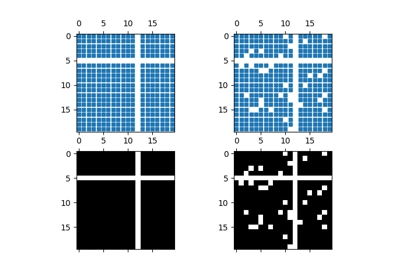matplotlib.pyplot.spy¶

matplotlib.pyplot.spy(Z, precision=0, marker=None, markersize=None, aspect='equal', **kwargs)[source]

Plot the sparsity pattern on a 2-D array.

spy(Z) plots the sparsity pattern of the 2-D array Z.

Parameters: Z : sparse array (n, m) The array to be plotted. precision : float, optional, default: 0 If precision is 0, any non-zero value will be plotted; else, values of $$|Z| > precision$$ will be plotted. For scipy.sparse.spmatrix instances, there is a special case: if precision is 'present', any value present in the array will be plotted, even if it is identically zero. origin : ["upper", "lower"], optional, default: "upper" Place the [0,0] index of the array in the upper left or lower left corner of the axes. aspect : ['auto' | 'equal' | scalar], optional, default: "equal" If 'equal', and extent is None, changes the axes aspect ratio to match that of the image. If extent is not None, the axes aspect ratio is changed to match that of the extent. If 'auto', changes the image aspect ratio to match that of the axes. If None, default to rc image.aspect value. Two plotting styles are available: image or marker. Both are available for full arrays, but only the marker style works for :class:scipy.sparse.spmatrix instances. If *marker* and *markersize* are *None*, an image will be returned and any remaining kwargs are passed to :func:~matplotlib.pyplot.imshow; else, a :class:~matplotlib.lines.Line2D object will be returned with the value of marker determining the marker type, and any remaining kwargs passed to the :meth:~matplotlib.axes.Axes.plot method. If *marker* and *markersize* are *None*, useful kwargs include: * *cmap* * *alpha*